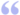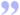# Nasal Operators

Nasal has support for various operators, both mathematical (add, subtract, multiply, and divide) and programming-specific (assignment, concatenation, control-flow, etc.). Most operators are in infix notation – that is, the operator is in the middle of its operands as in "3 + 5". As mentioned, Nasal supports the four basic math operators as +, -, *, and / (power is not available as ** (Python) or ^ (Visual basic), but rather is done in the math namespace as: math.pow(base, exponent)). For other math ones, assignment is just a single equals sign (=, as in var pi = 3.14) and equality comparison is double-equals (==, as in 3 == 3). Relative comparisons such as less-than (<), greater-than (>), less-than-or-equal-to (<=), and greater-than-or-equal-to (>=) also can be used (see Howto:Add a new binary operator to Nasal for an example of adding another comparison operator: the Perl "spaceship" operator, <=>). When operating on two variables using these operators (excepting assignment), Nasal uses a notion of "scalars" if applicable. Instead of the mathematical notion of scalars as non-vectors, a scalar in programming is defined as a number or numeric string – essentially duplicity of datatypes available to represent a number. This allows a string to also represent a number, which makes, e.g., parsing a number out of a file or string very easy, since the programmer doesn't have to think about the conversion. According to Andy (the creator of Nasal):Like perl, and unlike everything else, nasal combines numbers and strings into a single "scalar" datatype. No conversion needs to happen in user code, which simplifies common string handling tasks. — Andy RossThis presents a problem when you need to concatenate two or more numeric strings (which is often done with the same operator as addition). If the + operator sees two numeric strings, it will add them as numbers, thus "67" + "75" == 142 (resulting in a number), when one might want the string "35". Andy had to introduce a new operator to do concatenation, like the . operator in PHP, and that was the tilde (~). Here's some examples showing the difference:

```"88" + "88" == 176; # number
88 + "88" == "88" + 88; # same
"88" ~ "88" == "8888"; # string
88 ~ 88 == "8888"; # string as well
"88" ~ 88 == 88 ~ "88"; # same```

## Conditionals

Readers familiar with C/C++ will be familiar with the "ternary" operator for control-flow within an expression:

```# (condition) ? condition_true_expr1 : condition_false_expr2
x = reverse ? -x : x; # if (reverse) x = -x else x = x```

This is an expression that evaluates the first expression if the condition is true, or if it is false it evaluates the second expression. In most languages, this is an alternative to the if statement, which cannot be used as a value since it is a statement (in Nasal an if/else block is actually an expression, with the else value defaulting to nil if there is no else, so it is possible but rather ugly). The Python equivalent of the ternary-operator is: expr1 if (condition) else expr2.

Nasal also includes the boolean short-circuit operators "or" and "and" as keywords not symbols (not || and &&), like in Python. Unary negation is still ! like C/C++ (Python, however, does it as "not"). One of the uses for these is as a reduction of if statements:

```condition and setprop("/sim/gui/loaded", 1); # if (condition) setprop("/sim/gui/loaded", 1)
condition or setprop("/sim/gui/exited", 1); # if (!condition) setprop("/sim/gui/exited", 1) -- negated case of above```

Beware! if{}else{} does not (quite) work in this way:

`condition and setprop("/sim/gui/loaded", 1) or setprop("/sim/gui/exited", 1);`

"or" is of higher precedence than "and", so that is equivalent to:

`(condition and setprop("/sim/gui/loaded", 1)) or setprop("/sim/gui/exited", 1);`

Doesn't it seem silly to be including the return of setprop() in the value of the left expression, which then can influence the execution of the second piece of code? In particular:

```var hash = {};
!contains(hash, "loopid") and hash.loopid = 0 or hash.loopid += 1;```

Which evaluates like this:

1. hash is empty, so contains(hash, "loopid") is false, and !contains(...) is thus true, so
2. the right-hand side of and is tried, which is assignment to 0, and since the value of assignment is the right-hand side, it produces 0 aka false.
3. since the result of false and false is false (which is the left side of the or), the right side of the or is tried (!) as per short-circuit rules.
4. that means hash.loopid += 1 is evaluated – wait, didn't we want to not evaluate this?

This counter-example proves that using "... and ... or ..." isn't quite the same thing as using "if (...) ... else ..." expression (which is better done using the ternary operator, "... ? ... : ...), which can be a dangerous bug, so the rule is: don't use this.

## Slicing and indexing

 Note  As of 27 May 2014, the syntax expr[] is no longer valid and considered an error. It is neither a slice nor an index expression, although it utilized postfix brackets.

In Nasal, vectors can be sliced (i.e. a new vector is constructed from indexes of an existing vector) and strings, hashes, and vectors can be indexed/subscripted. For an overview of slicing see vector slicing.

Indexing works in different ways for the different types. For strings indexing returns a value equal to the value of the character at the position. For hashes it looks up the key in the hash directly, ignoring the parents vector, and returns nil if it is not found; this treats numbers and strings as different types of keys. For vectors, the value at the index of the array is retrieved, if it is valid. Some examples:

```var vec = [0,1,2,3];
vec == 0; vec == 1;
vec[-1] == 3; # last element
vec[-2] == 2; # next-from-last element

var hash = {
-1: 0,
"-1": 1,
hello: "hi",
parents: [{ find_me_here: 42 }]
};
hash[-1] == 0; # look up the number -1 in the hash
hash["-1"] == 1; # look up the string (!) "-1" in the hash
hash[-1~""] == 1; # same thing -- makes a string
hash.hello == "hi"; # find the member hello in the hash
hash["hello"] == "hi"; # approximately the same, but using an index expression
hash.find_me_here == 42; # find the member find_me_here in the hash, or actually its parent
hash["find_me_here"] == nil; # but it cannot be found with an index expression, because its parent isn't searched
hash.parents["find_me_here"] == 42; # but we can always look for the specific parent's member```

## Semantics

Some insights on the workings of various common operations.

### Equality

Here are the rules for checking equality:

• If both are scalars:
• If both are numeric: check if they are numerically equal (converting to numbers first).
• Else: check if they are equal as strings.
• Else compare id's (items only equal themselves, e.g. nil == nil while [] != [] because they are different vectors with different id's).

### Boolean evaluation

Rules for checking if a value is "true" or "false":

• If nil: return false
• If numeric:
• If 0: return false
• Else: return true
• Else: error "non-scalar in boolean context" (applies to hashes, functions, vectors, and ghosts)

## Table

Here's a summary of operators, in no particular order:

Symbol Operates on... Evaluates to...
+ and - and / and * Numeric scalars A number
~ Scalars A string
?: Three "simple" expressions: condition, expr1, and expr2 expr1 if the condition is true, else expr2
or Two "simple" expressions The first expression, if it evaluates to true, else the second
and Two "simple" expressions The first expression, if it evaluates to false, else the second